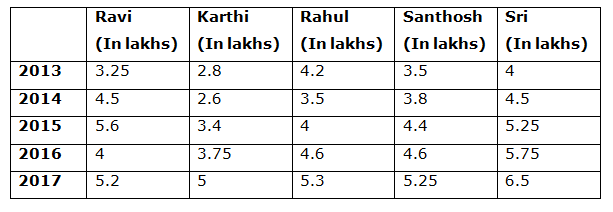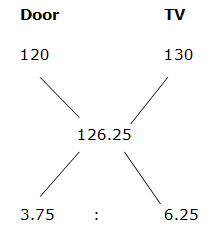# CWC/FCI Prelims 2019 – Quantitative Aptitude Questions (Day-29)

Dear Readers, Exam Race for the Year 2019 has already started, To enrich your preparation here we are providing new series of Practice Questions on Quantitative Aptitude – Section for CWC/FCI Exam. Aspirants, practice these questions on a regular basis to improve your score in aptitude section. Start your effective preparation from the right beginning to get success in upcoming CWC/FCI Exam.

[WpProQuiz 5744]

1) After 2 years, A’s age is equal to the present age of B. 4 years ago, C’s age is thrice the age of B. Find the A’s present age, if the present age of C is 22 years?

a) 12 years

b) 8 years

c) 15 years

d) 17 years

e) None of these

2) In a pack of 52 cards, two cards are taken out randomly. What is the probability that both are Jack cards?

a) 34/93

b) 1/221

c) 5/134

d) 27/97

e) None of these

3) A man buys a door and TV set for Rs. 40000. If he sells the doors at 20% profit and the TV set at Rs. 30 % profit then he earns a total profit of Rs. 10500. Find the selling price of a TV set?

a) 30000

b) 31500

c) 32500

d) 34000

e) None of these

4) The salary of A is 20 % less than B and B’s salary is 20 % more than C. If the sum of the salaries of A and B is Rs. 32400, then find the salary of C?

a) 17000

b) 15000

c) 19000

d) 21000

e) None of these

5) The simple interest on a sum of money is (1/9)th of the principle. The number of years is equal to the rate of interest per annum. Find the rate of interest per annum?

a) 3 1/3 %

b) 5 ¾ %

c) 4 ½ %

d) 6 3/5 %

e) None of these

Directions (6 – 10): Study the following information carefully and answer the given questions:

Following table shows the total annual profit of 5 business man in a certain state in different years.6) The total annual profit of Karthi and Rahul in the year 2015 and 2017 together is approximately what percentage of total annual profit of Ravi and Sri in the year 2013 and 2016 together?

a) 104 %

b) 118 %

c) 135 %

d) 96 %

e) 88 %

7) Find the average annual profit of Santhosh in all the given years together?

a) 92 lakhs

b) 78 lakhs

c) 36 lakhs

d) 31 lakhs

e) None of these

8) Find the ratio between the total annual profit of Ravi in the year 2013, 2015 and 2017 together to that of Rahul in the year 2014, 2015 and 2016 together?

a) 115 : 133

b) 281 : 242

c) 212 : 279

d) 338 : 445

e) None of these

9) Find the difference between the total annual profits of the given business man together in the year 2015 to that of 2017?

a) 2 lakhs

b) 4 lakhs

c) 6 lakhs

d) 8 lakhs

e) None of these

10) Which person has the third lowest total annual salary of all the given years together?

a) Rahul

b) Ravi

c) Karthi

d) Santhosh

e) Sri

After 2 years, A’s age = Present age of B

C’s present age = 22

4 years ago, C = 3*B

18 = 3*B

B’s age 4 years ago = (18/3) = 6

B’s present age = 10

After 2 years, A’s age = 10

A’s present age = 8 years

n(S) = 52C2

n(E) = 4C2

P(E) = n(E)/n(S) = 4C2/ 52C2

= > (4*3)/(52*51)

= > 1/221

Let the cost price of door and TV set be x and y respectively,

X + y = 40000 —à (1)

(20/100)*x + (30/100)*y = 10500

2x + 3y = 105000 —à (2)

By solving the equation (1) and (2), we get,

X = 15000, y = 25000

The selling price of TV set = 25000*(130/100) = Rs. 32500

(Or)

Percentage of total profit = (10500/40000)*100 = 26.25 %= > 375: 625

= > 3: 5

8’s = 40000

1’s = 5000

Cost price of TV set = 5’s = Rs. 25000

Selling price of TV set = 25000*(130/100) = Rs. 32500

The ratio of Salary of A and B = 80: 100 = 4: 5

The ratio of Salary of B and C = 120: 100 = 6: 5

The ratio of Salary of A, B and C = 24: 30: 25

54’s = 32400

1’s = 600

The salary of C = 25’s = Rs. 15000

1. I = Pnr/100

(1/9)*P = P*r2/100 [n = r]

(1/9) = r2/100

r2 = 100/9 = 10/3 = 3 1/3 %

Rate of interest = 3 1/3 %

Direction (6-10) :

The total annual profit of Karthi and Rahul in the year 2015 and 2017 together

= > 3.4 + 4 + 5 + 5.3 = 17.7 lakhs

The total annual profit of Ravi and Sri in the year 2013 and 2016 together

= > 3.25 + 4 + 4 + 5.75 = 17 lakhs

Required % = (17.7/17)*100 = 104 %

The average annual profit of Santhosh in all the given years together

= > (3.5 + 3.8 + 4.4 + 4.6 + 5.25)/5 = 21.55/5

= > 4.31 lakhs

The total annual profit of Ravi in the year 2013, 2015 and 2017 together

= > 3.25 + 5.6 + 5.2 = 14.05 lakhs

The total annual profit of Rahul in the year 2014, 2015 and 2016 together

= > 3.5 + 4 + 4.6 = 12.1 lakhs

Required ratio = 14.05 : 12.1 = 1405 : 1210

= > 281 : 242

The total annual profits of the given business man together in the year 2015

= > 5.6 + 3.4 + 4 + 4.4 + 5.25 = 22.65 lakhs

The total annual profits of the given business man together in the year 2017

= > 5.2 + 5 + 5.3 + 5.25 + 6.5 = 27.25 lakhs

Required difference = 27.25 – 22.65 = 4.6 lakhs

Ravi = > 3.25 + 4.5 + 5.6 + 4 + 5.2 = 22.55 lakhs

Karthi = > 2.8 + 2.6 + 3.4 + 3.75 + 5 = 17.55 lakhs

Rahul = > 4.2 + 3.5 + 4 + 4.6 + 5.3 = 21.6 lakhs

Santhosh = > 3.5 + 3.8 + 4.4 + 4.6 + 5.25 = 21.55 lakhs

Sri = > 4 + 4.5 + 5.25 + 5.75 + 6.5 = 26 lakhs

Rahul has third lowest total annual salary.